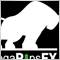• Übersicht
• Bewertungen (1)
• Diskussion (6)

# Close on floating amount

• Created to help traders to manage the risk.
• User introduces a positive and a negative floating amount.
• When account floating hits one of the two values introduced, all opened orders are closed.
• Of course, you can close your orders manually at any moment.
• You can change the parameters values stopping and restarting the EA.
• When EA is started, all opened orders apply to calculate the floating.

### EA parameters

• ProfitFloatingAmount- a number greater than zero. It's the positive floating to close all opened orders.
• LossFloatingAmount- a number less than zero. It's the negative floating to close all opened orders.

Example 1

• User introduces 3000 as ProfitFloatingAmount parameter and -2000 as LossFloatingAmount parameter.
• User has 4 opened orders with a current floating of 2500.
• Then, floating hits 3000 and all opened orders are closed.

Example 2

• User introduces 3000 as ProfitFloatingAmount parameter and -2000 as LossFloatingAmount parameter.
• User has 2 opened orders with a current floating of -1000.
• Then, floating hits -2000 and all opened orders are closed.327
2019.03.25 11:38

Der Benutzer hat keinen Kommentar hinterlassen